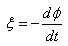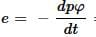NEET  >  Test: Faraday’s Laws of Induction

# Test: Faraday’s Laws of Induction

Test Description

## 10 Questions MCQ Test Physics Class 12 | Test: Faraday’s Laws of Induction

Test: Faraday’s Laws of Induction for NEET 2023 is part of Physics Class 12 preparation. The Test: Faraday’s Laws of Induction questions and answers have been prepared according to the NEET exam syllabus.The Test: Faraday’s Laws of Induction MCQs are made for NEET 2023 Exam. Find important definitions, questions, notes, meanings, examples, exercises, MCQs and online tests for Test: Faraday’s Laws of Induction below.
Solutions of Test: Faraday’s Laws of Induction questions in English are available as part of our Physics Class 12 for NEET & Test: Faraday’s Laws of Induction solutions in Hindi for Physics Class 12 course. Download more important topics, notes, lectures and mock test series for NEET Exam by signing up for free. Attempt Test: Faraday’s Laws of Induction | 10 questions in 10 minutes | Mock test for NEET preparation | Free important questions MCQ to study Physics Class 12 for NEET Exam | Download free PDF with solutions
 1 Crore+ students have signed up on EduRev. Have you?
Test: Faraday’s Laws of Induction - Question 1

### A magnet is moved towards the coil (i) quickly, (ii) slowly, the induced emf is​

Detailed Solution for Test: Faraday’s Laws of Induction - Question 1

When a magnet is moved towards the coil quickly, the rate of change of flux is larger than that if the magnetic field is moved slowly, thus larger emf is induced due to quick movement of the coil.

Test: Faraday’s Laws of Induction - Question 2

### The magnetic flux linked with a coil is changed from 1 Wb to 0.1 Wb in 0.1 second. The induced emf is​

Detailed Solution for Test: Faraday’s Laws of Induction - Question 2

Change n in magnetic flux Δϕ=0.1−1=−0.9 Weber
Time taken  Δt=0.1 sec
So, emf induced in the coil  E=− Δϕ​/Δt
⟹ E=− (−0.9)​/ 0.1=9 Volts
Hence option C is the correct answer.

Test: Faraday’s Laws of Induction - Question 3

### The principle of electromagnetic induction is used in which of the following phenomenon

Detailed Solution for Test: Faraday’s Laws of Induction - Question 3

In hydroelectricity the power of a turbine depends on the size of the water drop and the quantity of water flowing through the turbine. The water propels the turbine, which in turn powers an electric generator, which produces electricity under the principle of electromagnetic induction.

Test: Faraday’s Laws of Induction - Question 4

Which of the following law’s gives the direction of induced emf?

Detailed Solution for Test: Faraday’s Laws of Induction - Question 4

Lenz’s law is used for determining the direction of induced current.
Lenz’s law of electromagnetic induction states that the direction of induced current in a given magnetic field is such that it opposes the induced change by changing the magnetic field.
Following is the formula of Lenz’s law:
ϵ=−N (∂ϕB/∂t)
Where,

• ε is the induced emf
• ∂ΦB is the change in magnetic flux
• N is the number of turns in the coil

Lenz’s law finds application in electromagnetic braking and in electric generators

Test: Faraday’s Laws of Induction - Question 5

In the expression, what is the significance of negative sign?

Detailed Solution for Test: Faraday’s Laws of Induction - Question 5

The negative sign signifies that the induced emf always opposes any change in the magnetic flux.

Test: Faraday’s Laws of Induction - Question 6

Magnetic flux φ in Weber in closed circuit of resistance 15 ohm varies with time t sec as φ = 2t² – 3t + 1. The magnitude of induced emf at t = 0.25 sec is

Detailed Solution for Test: Faraday’s Laws of Induction - Question 6= 4t-3
At t = 0.25s
e= 2V

Test: Faraday’s Laws of Induction - Question 7

If the North Pole of the bar magnet is introduced into a coil at the end A which of the following statements correctly depicts the phenomenon taking place.

Detailed Solution for Test: Faraday’s Laws of Induction - Question 7

According to Lenz’s law the end A becomes the North Pole to oppose the motion of the magnet.
Hence, option B is correct.

Test: Faraday’s Laws of Induction - Question 8

In which of the following cases with a bar magnet and the coil no induced emf is induced.

Detailed Solution for Test: Faraday’s Laws of Induction - Question 8

This is because induced emf directly depends on relative motion between the magnet and coil, but if there is no relative motion between them, there will be no emf induced.

Test: Faraday’s Laws of Induction - Question 9

The role of inductance is equivalent to:

Detailed Solution for Test: Faraday’s Laws of Induction - Question 9

Self induction is that phenomenon in which a change in electric current in a coil produces an induced emf in the coil itself.
Now, it is also known as inertia of electricity as for if we were to change electric current through a current carrying coil it will tend to oppose any further change in its emf. This is similar to inertial behavior in mechanics where bodies in either rest or motion tend to oppose any change in their state. Here mass is the inertial property analogous to self inductance.

Test: Faraday’s Laws of Induction - Question 10

A coil of 100 turns is pulled in 0.04 sec. between the poles of a magnet, if its flux changes from 40 x 10-6 Wb per turn to 10-5 Wb per turn, then the average emf induced in the coil is​

Detailed Solution for Test: Faraday’s Laws of Induction - Question 10

Emf = - N(∆magnetic flux)/∆t
N=100turns
∆magnetic flux= -30×10-6Wb
∆t = 0.04sec
Emf = -(100turns)(-30×10-6)/(0.04sec)
Emf = 0.075V

## Physics Class 12

157 videos|452 docs|213 tests
 Use Code STAYHOME200 and get INR 200 additional OFF Use Coupon Code